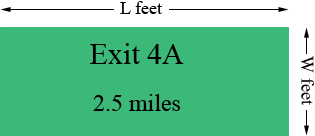SEARCH HOMEMath Central Quandaries & QueriesQuestion from Stephanie, a student: A standard bill on a highway has a perimeter of 14 feet. The length of the bill board is 6 feet greater than triple the width. What is the length? what is the width?Stephanie,

Draw a diagram!I let the length be L feet and the width W feet. Since the perimeter is 14 feet

L + W = 7 feet.

The problem says "The length of the bill board is 6 feet greater than triple the width." If the width is W feet what is triple the width? What is 6 feet more that triple the width? This value is L. Substitute this value for L in the equation above and solve for W.

Penny

Stephanie,

Do you know the formula for the perimeter of a rectangle of lenght L and width W?? If not, draw one and count how many "long edges" and how many "wide edges" make up the entire boundary.

the length of the bill board is 6 feet greater than triple the width.

If the width is W, what is triple the width?

What is 6 more than triple the width?

You should now have two linear equations, each of the form

? L + ? W = ? or ?L = ?W + ? or ?W = ?L + ?

(each question mark stands for something different.)

Now try to use algebra to turn one of these equations into an
equation of the form

L = aW + b
or
W = aL + b.

(You can do anything to one side provided you do it to the other as well. So if one equation was (it isn't)

3L + 6W = 21

you could divide both sides by 3 to get

L + 2W = 7

and subtract 2W from both sides to get

L = 7 - 2W.

Now substitute [in this case; you'll do it differently] (7-2W) for L inthe other equation to get an equation with only W's in. Solve this in the same way, using algebra to put it into a form like

W=4

Finally, plug that back in to get a value for L.

Good hunting!
-RDMath Central is supported by the University of Regina and The Pacific Institute for the Mathematical Sciences.